## Hydraulic Pressure Multiplication

Written by Drenchenator
Last updated on 2007-10-05Piston water guns can benefit from an important application of hydraulics: force multiplication from Pascal's Principle. In most piston water guns, the user inputs some force to a piston which in turn physically moves the water through a nozzle, making a stream.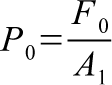Whatever force the user inputs creates a pressure in the hydraulic fluid used in this setup. By the definition of pressure, the pressure equals the force over the area of the piston that exerts this force into the fluid.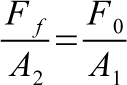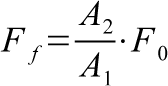Fluids carry pressures. The hydraulic fluid carries the pressure to the second piston, creating a larger force. However, the larger force will only make gun easy to use because the force will be multiplied. The gun needs a third piston to perform better.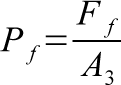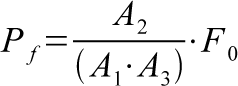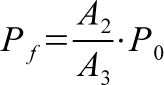The new force is applied to a third, final piston. This piston is smaller than the second but larger than the first. Now, a higher pressure can be achieved because the new force is applied over a different area.

But how are the force and pressure increased? Is the energy conserved?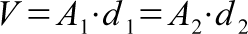The hydraulic fluid volume dictates the size of the size of the first and second pistons. This volume can be taken as the products of their respective areas and distances traveled.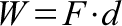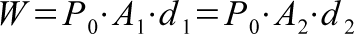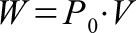Work or energy is the product of a force and a distance. In a sense, it is the energy received from a force covering a distance. Substitution shows that the work for each piston equals the pressure by the force by the distance the piston travels. This equation also serves as a continuity equation for the energy. Since the volume is set and the products of area and distance are equal, the work done on each piston is the same. The first piston receives less force but must travel a greater distance. The second piston receives more force but travels less distance. Energy is conserved.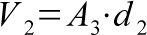Energy is also conserved for the third piston. Its work equals its received force by its traveled distance. However, the volume is different in this case. Since this piston can only travel with the second, its distance traveled is the same as the second. Therefore the volume of water it can push out is the product of the third piston's area and the second piston's distance.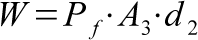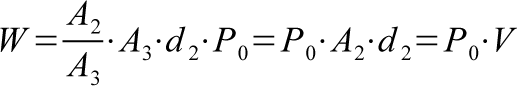Substitution and simplication show that energy is conserved. The work done to it is the same as the other two pistons.

< http://www.sscentral.org/physics/multiplication.html >